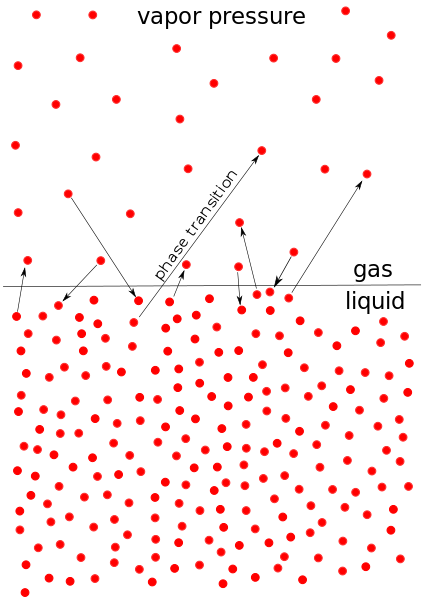## Vapor Pressure of Nonelectrolyte Solutions

#### Learning Objective

• Calculate the vapor pressure of a nonelectrolyte solution using Raoult’s law

#### Key Points

• The vapor pressure of a system is a measure of the molecules’ tendency to escape into the gas phase.
• Vapor pressure is a colligative property, meaning that the amount it is increased or decreased is directly related to the amount of solute present in the system.
• Raoult’s law states that the partial vapor pressure of each component of an ideal mixture of liquids is equal to the vapor pressure of the pure component multiplied by its mole fraction in the mixture

#### Terms

• vapor pressureThe pressure that a vapor exerts, or its partial pressure if it is mixed with other gases.
• colligative propertyA property of solutions that is directed by the ratio of solute to solvent, regardless of the identity of the solute.
• Raoult’s law
• raoult’s lawStates that the partial vapor pressure of each component of an ideal mixture of liquids is equal to the vapor pressure of the pure component multiplied by its mole fraction in the mixture

## Colligative Properties: Vapor Pressure

Vapor pressure is a measure of the tendency of a material to escape into the environment via gas. A substance that evaporates quickly has high vapor pressure and is referred to as a volatile substance. When a solute is added to a solvent, the vapor pressure decreases.Vapor pressureVapor pressure measures the particle transition from the liquid phase to the gas phase and vice versa.

## Lowering Vapor Pressure

The vapor pressure of a solvent is lowered by the addition of a non-volatile solute to form a solution. By definition, a non-volatile substance does not evaporate. This decrease in vapor pressure can be explained by using the entropy differences of the liquid and gas phases along with the position of dissolved particles after the addition of the solute.

## Entropy

When molecules transition from the liquid phase to the gas phase, entropy of the system increases. Entropy of the gaseous state is greater than the entropy of the liquid state because the gaseous molecules occupy a larger volume.

If the liquid solvent becomes “diluted” with solute, the entropy of the liquid state increases. Therefore, even though the gaseous state has a higher entropy, the difference in entropy between the two systems decreases. The decrease in entropy difference lowers the vapor pressure.

## Solute Particle Location

In a pure solvent, all of the liquid molecules on the surface can evaporate and transition to the gaseous phase. In a solution, some spots on the surface are occupied by solute molecules, so there is less space occupied by solvent molecules. Overall, less solvent will transition to the gaseous phase, resulting in a decrease in vapor pressure.

## Equilibrium Vapor Pressure Given by Raoult’s Law

Raoult’s law states that the vapor pressure of an ideal solution is dependent on the vapor pressure of the pure solvent and the mole fraction of the component present in the solution. For an ideal solution, equilibrium vapor pressure is given by Raoult’s law:

$p = p^{\star}_{\rm A} x_{\rm A}$

In this equation, $p^{\star}_{\rm A}$ is the vapor pressure of the pure solvent and $x_{\rm A}$ is the mole fraction of the solvent. This law allows us to calculate the vapor pressure of a given solution, and shows the influence of all of the molecules in the solution.

## Example

Calculate the vapor pressure of a solution consisting of 3 moles of a nonvolatile solute and 15 moles of water at 25 oC, given that the vapor pressure of pure water at 25 oC is 23.8 torr.

First, calculate the mole fraction of the solvent:

$x_{\rm A} = \frac{moles\ solvent}{total\ moles}$

$x_{\rm A} = \frac{15}{18} = 0.83$

Second, substitute into Raoult’s law and solve:

$p = p^{\star}_{\rm A} x_{\rm A}$

$p = 23.8\ torr \times 0.83$

$p = 19.8\ torr$